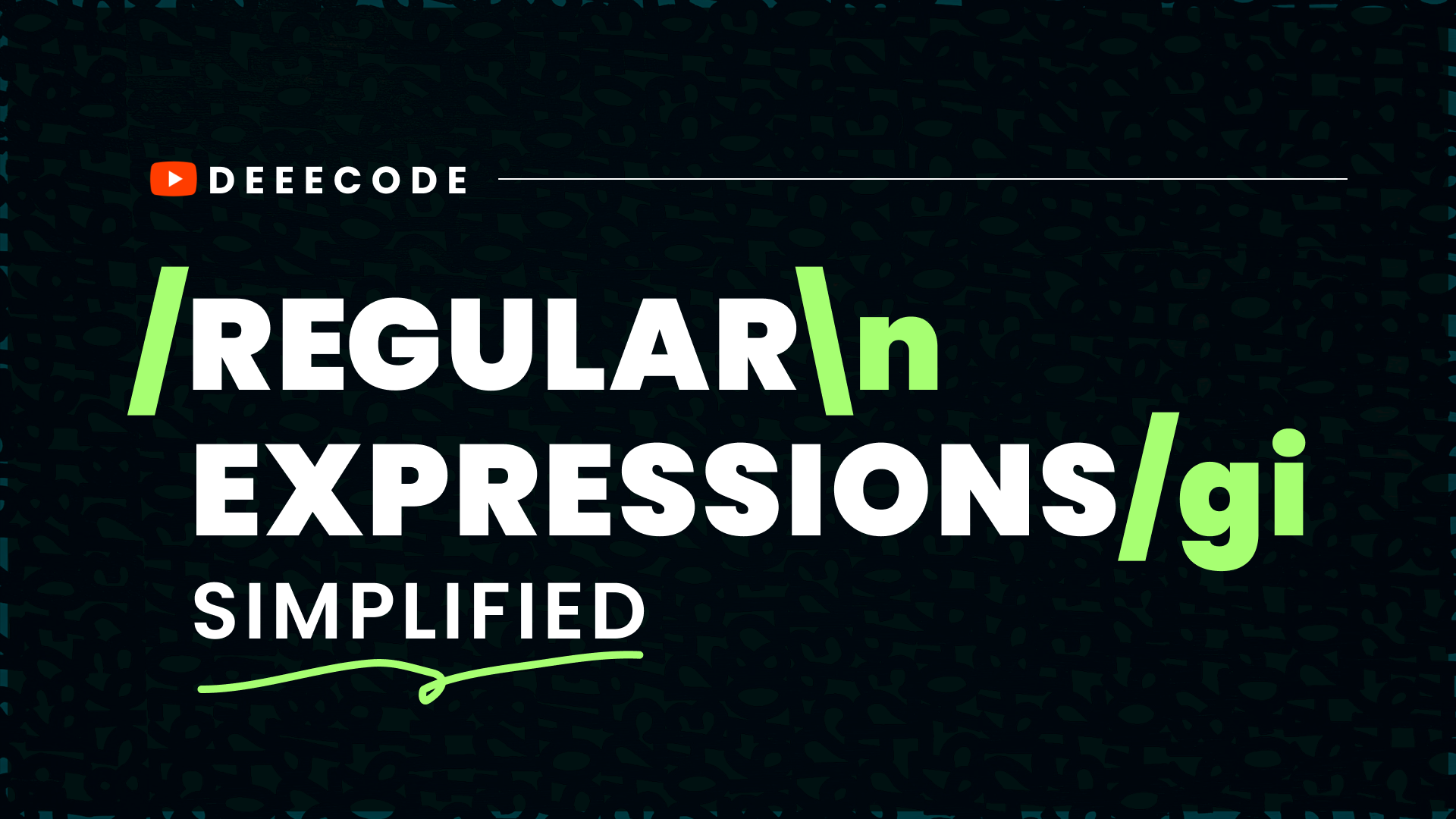# Mutability vs Immutability in Javascript

November 26th, 2019 | 2 mins read### What is Immutability

Immutability is an attribute which stands for unchangeable. When an object is immutable, it is either unable to change or would never be changed in it's existence.

### What is Mutability

On the other hand, this stands for changeable. Objects possessing this attribute would change at some point.

### TL,DR;

Try as much as possible to always work with immutable objects when coding.

## How do you know when an object is immutable or mutable?

In javascript, variables can store two major data types - `primitive` and `reference`.

• Primitive data types include `number`, `string`, `symbol`, `boolean`, `undefined` and `null`.
• Reference data types include `object`.

Primitive values are stored directly in the location that the variable accesses.

Reference values are stored in the variable location which is a pointer to a location in memory where the object is stored.

### Examples

• Immutability
``````let a = 15
let b = a
b = b - 1
console.log(a)
console.log(b)
// Output:
// 15
// 14``````

Variable `a` remains immutable, same as `b`. This is the same for other primitive type of variables.

• Mutability
``````let a = ["first", "second"]
let b = a
b.pop()
console.log(a)
console.log(b)
// Output
// ['first'];
// ['first'];``````

You notice that `a` is also affected? That's because it is a mutable object. The same situation goes to objects

``````let c = {
first: "javascript",
second: "language",
}
let d = c
d.first = "react"
console.log(c)
console.log(d)
// Output
// {first: 'react', second: 'language'}
// {first: 'react', second: 'language'}``````

Variable `c` is a reference variable and any other variable which it is assigned to only leaves a reference and not a new location for the variable. The examples above are simple instances but imagine having something like

``````let d = c;
// some  manipulations
let e = c;
// some manipulations
...``````

There would be unexpected results as the initial object which the developer thinks he is working with has been mutated. However, there are solutions to this.

## Making Immutable Objects

Our aim now is to assign a new location to object variables even when they may require values from previous objects.

### Array Solution

#### The `concat()` method

Back to our code above, we can define a new location to the `b` array by using the `concat` method.

``````let a = ["first", "second"]
let b = a.concat()
b.pop()
console.log(a)
console.log(b)
// Output
// ['first', 'second'];
// ['first'];``````

Note that you can add more arrays as parameters to the concat method. This has assigned a new location to `b` while retaining all values in `a`.
This used to be the usual way of creating new objects from previous ones but ES6 introduced a new method which solves the mutability problem.

#### the `spread` operator

Usage:

``````let a = ["first", "second"]
let b = [...a, "third", "fourth"]
b.pop()
console.log(a)
console.log(b)
// Output
// ['first', 'second'];
// ['first', 'second', 'third'];``````

`b` simply steals the values in `a` and adds new values but uses a new location in memory.

### Object Solution

#### `Object.assign()`

``````let c = {
first: "javascript",
second: "language",
}
let d = Object.assign({}, c)
d.first = "react"
console.log(c)
console.log(d)
// Output
// {first: 'javascript', second: 'language'}
// {first: 'react', second: 'language'}``````

This method (as used above) creates a new object, but assigns the values of `c` to included in it. With this, we get a new location in memory for `d` contents.

#### Using the `spread` operator

This operator can also be used for objects.

``````let c = {
first: "javascript",
second: "language",
}
let d = { ...c }
d.first = "react"
console.log(c)
console.log(d)
// Output
// {first: 'javascript', second: 'language'}
// {first: 'react', second: 'language'}``````

Similar to arrays, `d` steals the contents of `c` and is assigned to a new location.

I hope you have gotten to understand the concept of mutability and immutability, the side-effects (positive and negative) and how to control variables created.